# 第8周

## 十三、聚类(Clustering)

### 13.1 无监督学习：简介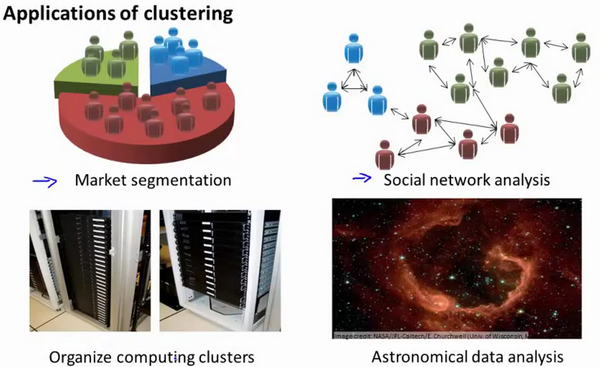### 13.2 K-均值算法

K-均值是最普及的聚类算法，算法接受一个未标记的数据集，然后将数据聚类成不同的组。

K-均值是一个迭代算法，假设我们想要将数据聚类成n个组，其方法为: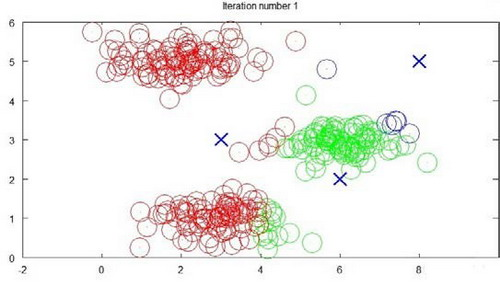$μ^1$,$μ^2$,...,$μ^k$ 来表示聚类中心，用$c^{(1)}$,$c^{(2)}$,...,$c^{(m)}$来存储与第$i$个实例数据最近的聚类中心的索引，K-均值算法的伪代码如下：

 ​xRepeat {​for i = 1 to m​c(i) := index (form 1 to K) of cluster centroid closest to x(i)​for k = 1 to K​μk := average (mean) of points assigned to cluster k​}

K-均值算法也可以很便利地用于将数据分为许多不同组，即使在没有非常明显区分的组群的情况下也可以。下图所示的数据集包含身高和体重两项特征构成的，利用K-均值算法将数据分为三类，用于帮助确定将要生产的T-恤衫的三种尺寸。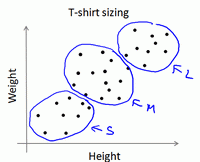### 13.3 优化目标

K-均值最小化问题，是要最小化所有的数据点与其所关联的聚类中心点之间的距离之和，因此 K-均值的代价函数（又称畸变函数 Distortion function）为：

$J(c^{(1)},...,c^{(m)},μ_1,...,μ_K)=\dfrac {1}{m}\sum^{m}_{i=1}\left\| X^{\left( i\right) }-\mu_{c^{(i)}}\right\| ^{2}$

### 13.4 随机初始化

1. 我们应该选择$K，即聚类中心点的个数要小于所有训练集实例的数量
2. 随机选择$K$个训练实例，然后令$K$个聚类中心分别与这$K$个训练实例相等

K-均值的一个问题在于，它有可能会停留在一个局部最小值处，而这取决于初始化的情况。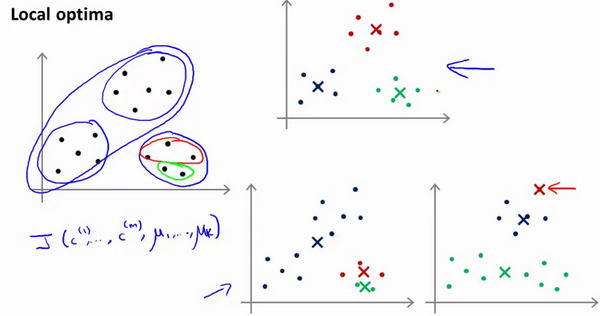### 13.5 选择聚类数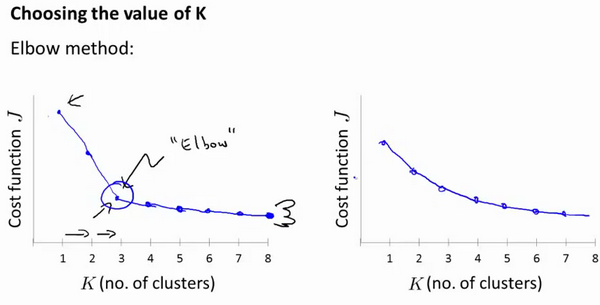1.相似度/距离计算方法总结

(1). 闵可夫斯基距离Minkowski/（其中欧式距离：$p=2$)

$dist(X,Y)={{\left( {{\sum\limits_{i=1}^{n}{\left| {{x}_{i}}-{{y}_{i}} \right|}}^{p}} \right)}^{\frac{1}{p}}}$

(2). 杰卡德相似系数(Jaccard)：

$J(A,B)=\frac{\left| A\cap B \right|}{\left|A\cup B \right|}$

(3). 余弦相似度(cosine similarity)：

$n$维向量$x$$y$的夹角记做$\theta$，根据余弦定理，其余弦值为：

$cos (\theta )=\frac{{{x}^{T}}y}{\left|x \right|\cdot \left| y \right|}=\frac{\sum\limits_{i=1}^{n}{{{x}_{i}}{{y}_{i}}}}{\sqrt{\sum\limits_{i=1}^{n}{{{x}_{i}}^{2}}}\sqrt{\sum\limits_{i=1}^{n}{{{y}_{i}}^{2}}}}$ (4). Pearson皮尔逊相关系数： ${{\rho }_{XY}}=\frac{\operatorname{cov}(X,Y)}{{{\sigma }_{X}}{{\sigma }_{Y}}}=\frac{E[(X-{{\mu }_{X}})(Y-{{\mu }_{Y}})]}{{{\sigma }_{X}}{{\sigma }_{Y}}}=\frac{\sum\limits_{i=1}^{n}{(x-{{\mu }_{X}})(y-{{\mu }_{Y}})}}{\sqrt{\sum\limits_{i=1}^{n}{{{(x-{{\mu }_{X}})}^{2}}}}\sqrt{\sum\limits_{i=1}^{n}{{{(y-{{\mu }_{Y}})}^{2}}}}}$

Pearson相关系数即将$x$$y$坐标向量各自平移到原点后的夹角余弦。

2.聚类的衡量指标

(1). 均一性：$p$

(2). 完整性：$r$

(3). V-measure:

$V = \frac{(1+\beta^2)*pr}{\beta^2*p+r}$

(4). 轮廓系数

$s(i) = \frac{b(i)-a(i)}{max\{a(i),b(i)\}}$

(5). ARI

$X=\{{{X}_{1}},{{X}_{2}},...,{{X}_{r}}\},Y=\{{{Y}_{1}},{{Y}_{2}},...,{{Y}_{s}}\}$

$X$$Y$的元素个数为：

$a=\{{{a}_{1}},{{a}_{2}},...,{{a}_{r}}\},b=\{{{b}_{1}},{{b}_{2}},...,{{b}_{s}}\}$$ARI=\frac{\sum\limits_{i,j}{C_{{{n}_{ij}}}^{2}}-\left[ \left( \sum\limits_{i}{C_{{{a}_{i}}}^{2}} \right)\cdot \left( \sum\limits_{i}{C_{{{b}_{i}}}^{2}} \right) \right]/C_{n}^{2}}{\frac{1}{2}\left[ \left( \sum\limits_{i}{C_{{{a}_{i}}}^{2}} \right)+\left( \sum\limits_{i}{C_{{{b}_{i}}}^{2}} \right) \right]-\left[ \left( \sum\limits_{i}{C_{{{a}_{i}}}^{2}} \right)\cdot \left( \sum\limits_{i}{C_{{{b}_{i}}}^{2}} \right) \right]/C_{n}^{2}}$

## 十四、降维(Dimensionality Reduction)

### 14.1 动机一：数据压缩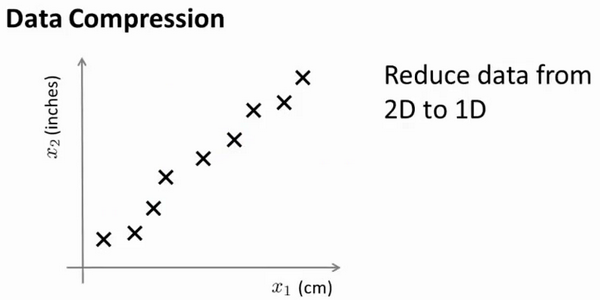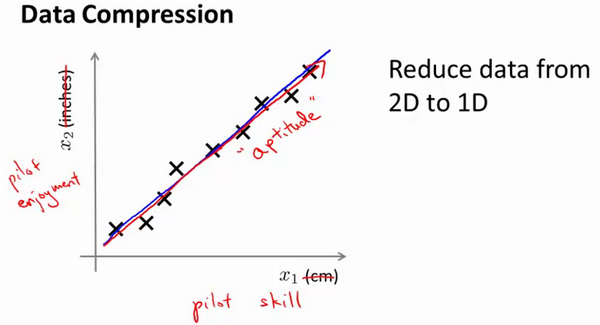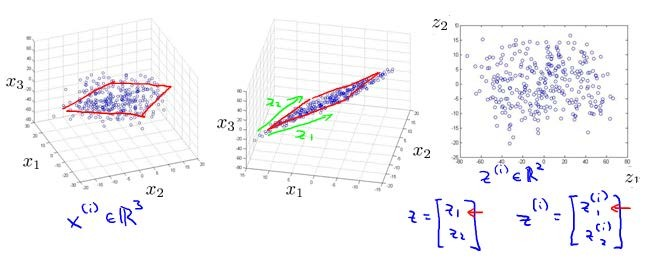### 14.2 动机二：数据可视化### 14.3 主成分分析问题

PCA中，我们要做的是找到一个方向向量（Vector direction），当我们把所有的数据都投射到该向量上时，我们希望投射平均均方误差能尽可能地小。方向向量是一个经过原点的向量，而投射误差是从特征向量向该方向向量作垂线的长度。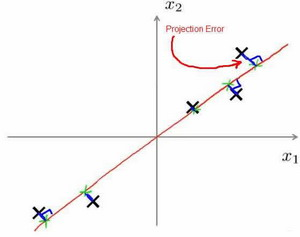PCA$n$个特征降维到$k$个，可以用来进行数据压缩，如果100维的向量最后可以用10维来表示，那么压缩率为90%。同样图像处理领域的KL变换使用PCA做图像压缩。但PCA 要保证降维后，还要保证数据的特性损失最小。

PCA技术的一大好处是对数据进行降维的处理。我们可以对新求出的“主元”向量的重要性进行排序，根据需要取前面最重要的部分，将后面的维数省去，可以达到降维从而简化模型或是对数据进行压缩的效果。同时最大程度的保持了原有数据的信息。

PCA技术的一个很大的优点是，它是完全无参数限制的。在PCA的计算过程中完全不需要人为的设定参数或是根据任何经验模型对计算进行干预，最后的结果只与数据相关，与用户是独立的。

### 14.4 主成分分析算法

PCA 减少$n$维到$k$维：

Octave 里我们可以利用奇异值分解singular value decomposition）来求解，[U, S, V]= svd(sigma)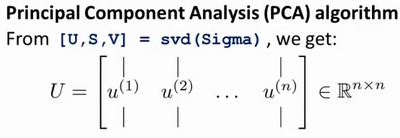$Sigma=\dfrac {1}{m}\sum^{n}_{i=1}\left( x^{(i)}\right) \left( x^{(i)}\right) ^{T}$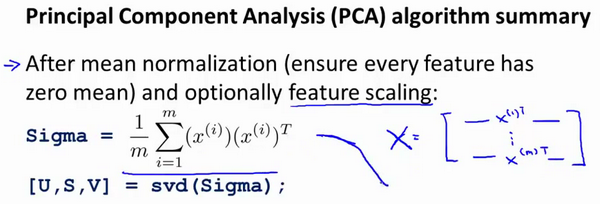### 14.5 选择主成分的数量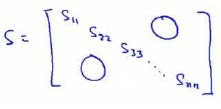### 14.6 重建的压缩表示PCA算法，我们可能有一个这样的样本。如图中样本$x^{(1)}$,$x^{(2)}$。我们做的是，我们把这些样本投射到图中这个一维平面。然后现在我们需要只使用一个实数，比如$z^{(1)}$，指定这些点的位置后他们被投射到这一个三维曲面。给定一个点$z^{(1)}$，我们怎么能回去这个原始的二维空间呢？$x$为2维，z为1维，$z=U^{T}_{reduce}x$，相反的方程为：$x_{appox}=U_{reduce}\cdot z$,$x_{appox}\approx x$。如图：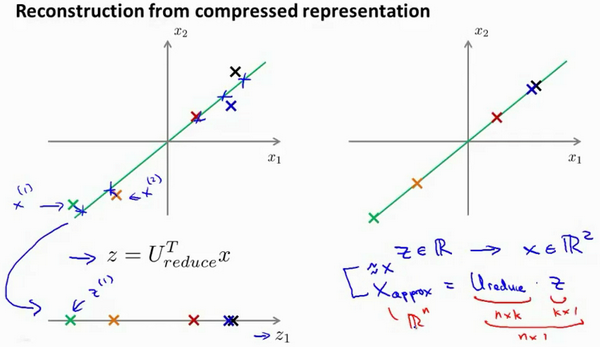### 14.7 主成分分析法的应用建议

1. 第一步是运用主要成分分析将数据压缩至1000个特征

2. 然后对训练集运行学习算法

1. 在预测时，采用之前学习而来的$U_{reduce}$将输入的特征$x$转换成特征向量$z$，然后再进行预测